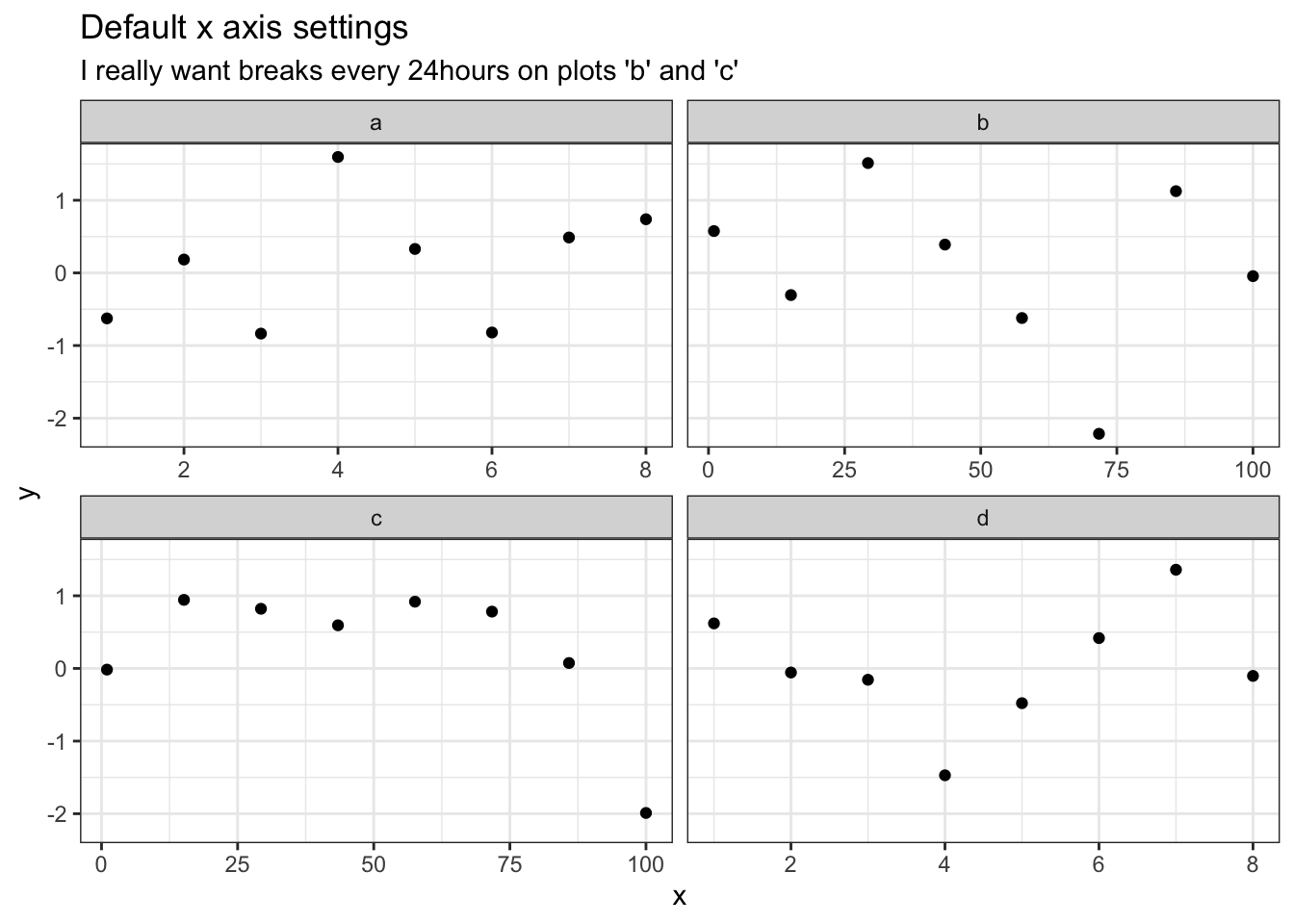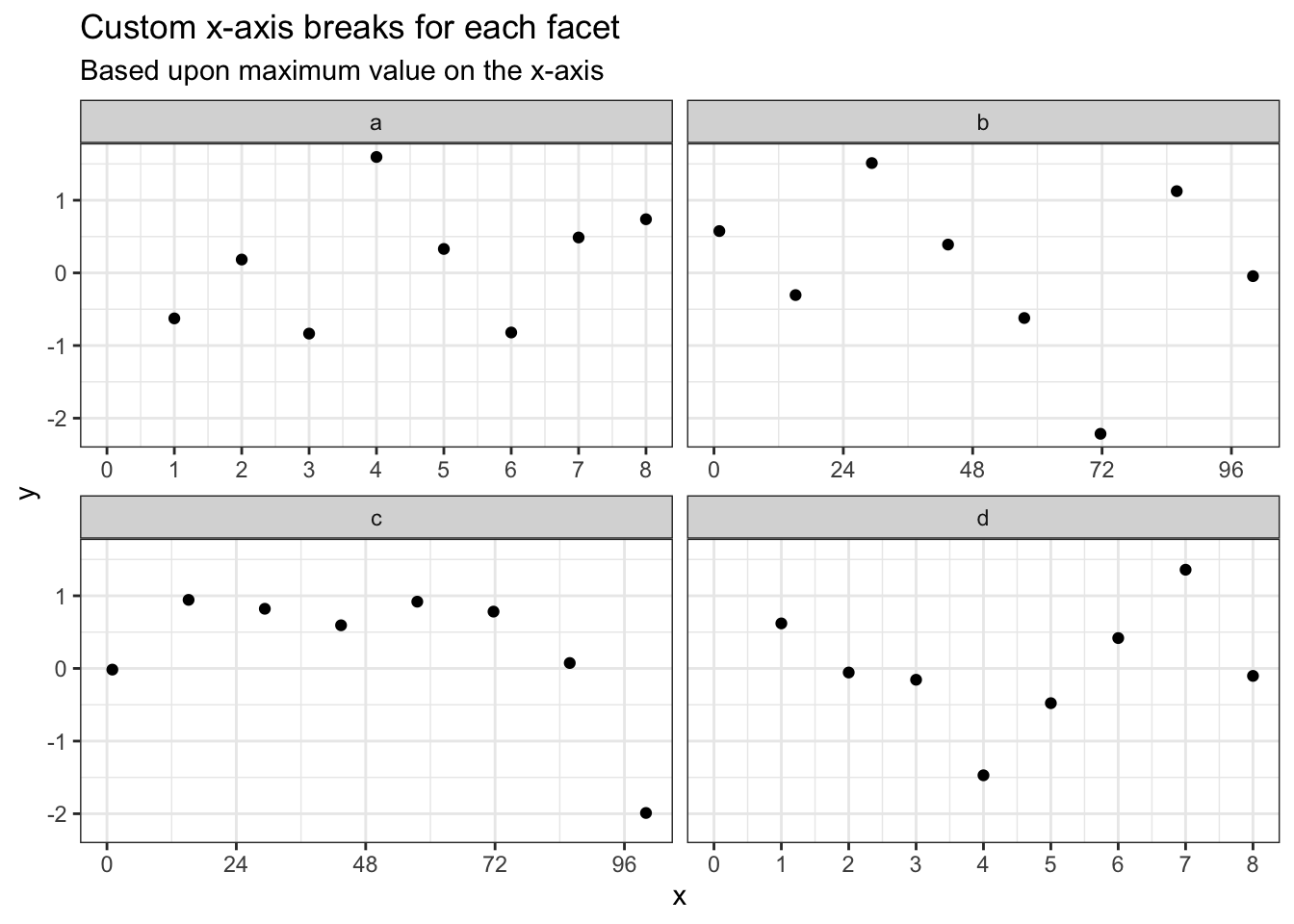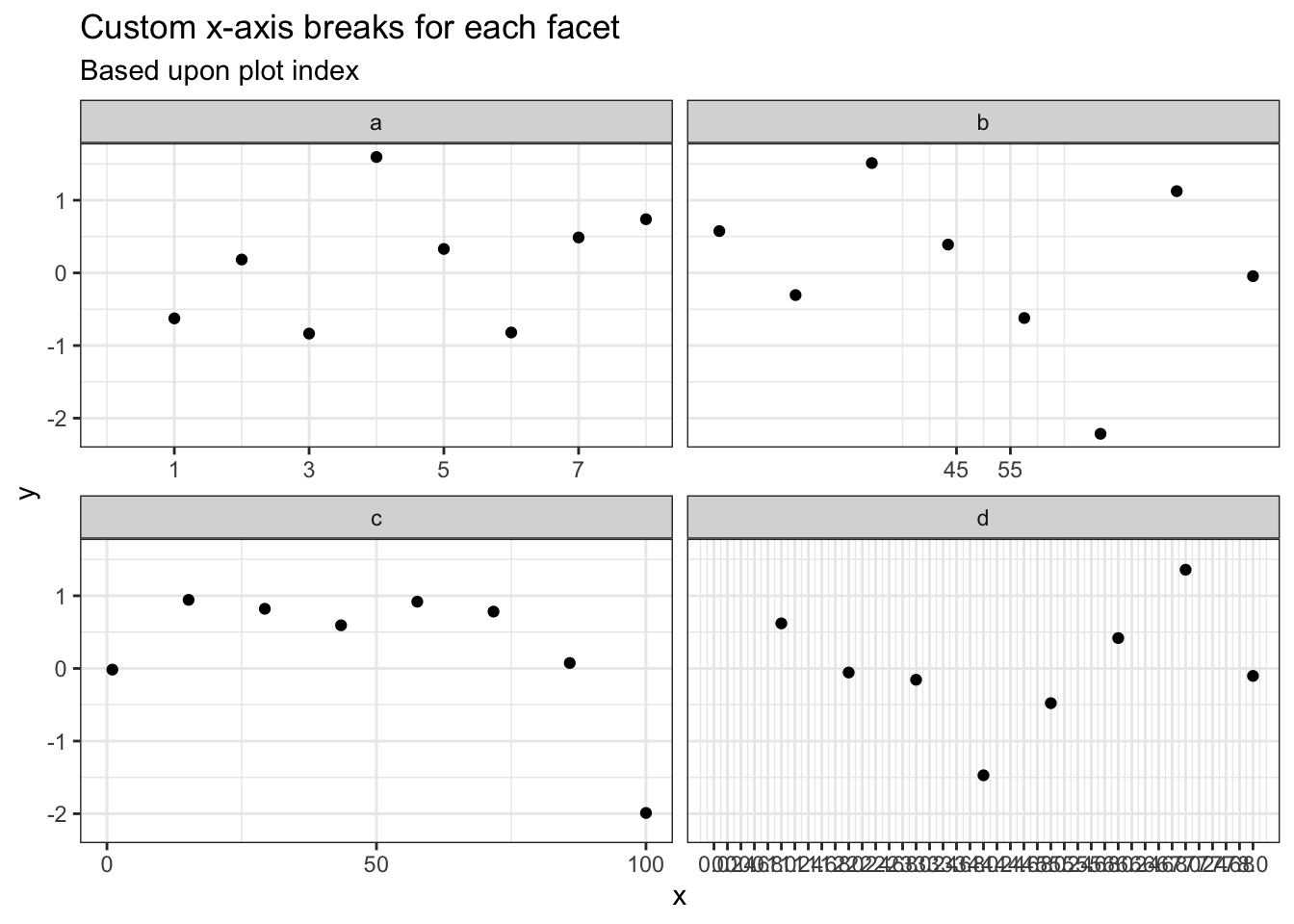# Custom axis breaks on facetted ggplot

I needed to create a facetted ggplot with custom x-axis breaks on every single plot.

The x-axis had units of hours and some plots spanned multiple days, and other spanned just a few hours. For the facets that spanned multiple days I wanted breaks at every 24 hours, but for the shorter times I needed breaks every hour.`ggplot2` allows you to use a function to set the `breaks` for the x-axis, so I could just check what the limits of the plot are and do two different things depending on the upper limit.

``````breaks_fun <- function(x) {
if (max(x) > 24) {
seq(0, 120, 24)
} else {
seq(0, 8, 1)
}
}

ggplot(df) +
geom_point(aes(x, y)) +
facet_wrap(~id, scales = 'free_x') +
theme_bw() +
scale_x_continuous(breaks = breaks_fun, limits = c(0, NA)) +
labs(
title = "Custom x-axis breaks for each facet",
subtitle = "Based upon maximum value on the x-axis"
)``````And then I could use a global variable to keep track of which plot is being addressed and customise x-axis breaks even further.

``````count <- 0
breaks_fun <- function(x) {
count <<- count + 1L
switch(
count,
c(1, 3, 5, 7, 9),
c(45, 55),
c(0, 50, 100),
seq(0, 8, 0.2)
)
}

ggplot(df) +
geom_point(aes(x, y)) +
facet_wrap(~id, scales = 'free_x') +
theme_bw() +
scale_x_continuous(breaks = breaks_fun, limits = c(0, NA)) +
labs(
title = "Custom x-axis breaks for each facet",
subtitle = "Based upon plot index"
)``````